### Home > A2C > Chapter 13 > Lesson 13.1.3 > Problem13-58

13-58.
1. Quickly sketch each of the following functions. Homework Help ✎

1. f(x) = (x − 1)2(x − 3)3(x − 5)2

2. g(x) = −(x − 1)2(x − 3)3(x − 5)2

3. Express g(x) in terms of f(x).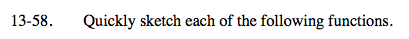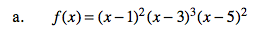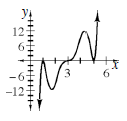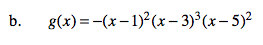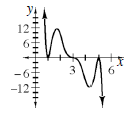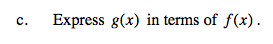g(x) = (?) · f(x)